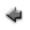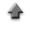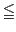previoushome

# Basic statistics

Basic statistics can be calculated from data. To start, click "Basic Statistics" tab on the application.
If you use the sample data, click here, and save it in the appropriate directory (or folder).

When you calculate the basic statistics for the sample data,

1. Click "Open data file", and specify the data file prepared according to the above instruction.
2. When clicking the "Show statistics" button bellow, the result is displayed.

## Data

1. Each label for data can be set in the first row in the data file. The label can not include spaces. Each label has to be delimited by space or tab.
2. Data must be inputted by normal width.
3. Input a numerical datum a line in the data file, change line, and input the datum of the following row similarly until the input of the last datum ends. Refer to the following examples, where x1, x2,...,xn are the numerical data.
 x1 x2 . . . xn
4. In addition, you can use the table data form in the data file. You may include the column data not used to calculate in the data file. In that case, the specification of the column used to calculate is needed at the line "Data columns" in the following "Input" instruction.

## Inputs

1. Click "Open data file", and specify the data file prepared according to the above instruction.
2. When the labels for data are used, check the "Data labels".
3. The column in the data file to be used to calculate the statistics can be specified at the line "Data columns". For instance, when the data to calculate the statistics are put at the fifth column in the data file, specify "5" at the line "Data columns", and check the "Data columns" box.
4. The range of the data to calculate the statistics can be specified at the line "Data range". In that case, specify the range of the data (the numerical values must be delimited by space), and check the "Data range" box. For instance, when you want to assume the range of the data x to be 0x15, specify "0 15".

## Outputs

1. A result is displayed when the "Show statistics" button is clicked.

Kazushi Neichi